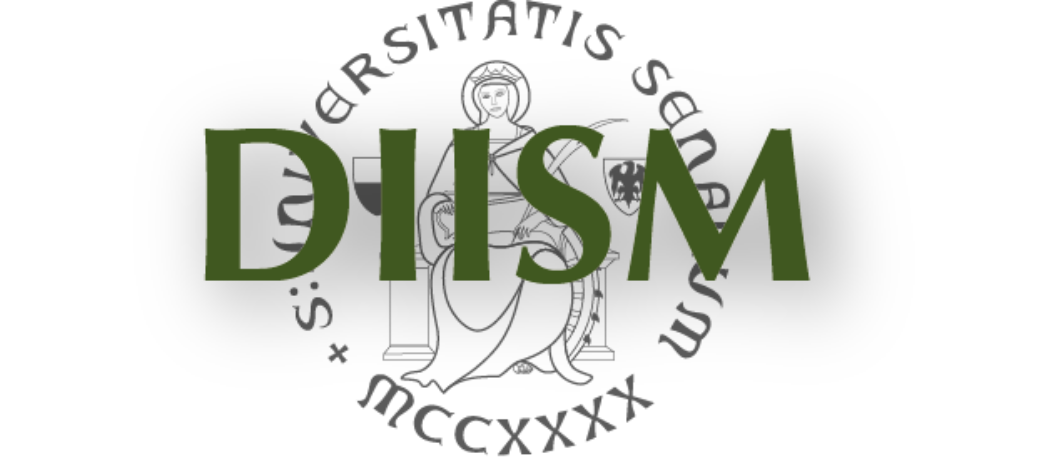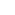DIPARTIMENTO DI INGEGNERIA DELL'INFORMAZIONEE SCIENZE MATEMATICHE UNIVERSITA' DEGLI STUDI DI SIENAPrivacy e Cookie policy

 EducationCorsi di studioInsegnamenti

InsegnamentoComplex dynamic systems a.a. 2013-2014 Corso Lm Ingegneria GestionaleLm Computer and Automation Engineering [RA] Anno 1 Semestre I Obiettivi The course presents an introduction to the theory of nonlinear dynamic systems, including bifurcation theory and qualitative analysis. Algorithms for the simulation of complex dynamics, such as limit cycle oscillations and chaotic behavior are presented. Several examples of complex systems in discrete and continuous time are tackled, with application to population dynamics, economic, ecological and biological systems. Specific objectives of the Course are: Modeling and analysis of complex nonlinear dynamical systems. Introduction of fundamental mathematical concepts for understanding and analyzing autonomous linear and non linear ordinary differential equations, including qualitative analysis. Criteria and theorems for the asymptotic stability of equilibria. Linearization and Hartman-Grobman theorem. Linear and nonlinear oscillations (limit cycles). Bifurcations: saddle-node, transcritical, pitchfork and Hopf bifurcations in continuous time, flip and period doubling bifurcations in discrete and continuous time, respectively. Bifurcation cascades and routes to chaos. Chaotic attractors and fractals. Simulation of nonlinear systems. Software tools for the analysis of complex systems: MATLAB, Content, Matcont. Analysis and simulation of complex systems in multidisciplinary fields: physical, biological, ecological and economic systems. Ore 48 Crediti 6 Link corso http://www.dii.unisi.it/~mocenni/compdynsys-teach.html Docente Chiara Mocenni
 Note The course includes theoretical lectures and practical laboratories. The course web site presents detailed information on program, teaching material and organization of the exams.
 Programma Complex dynamic systems 1. Continuous time systems - Linear and nonlinear differential equations - Vector fields, phase space and differential equations - Stability of stady states - Linearization of nonlinear systems - Oscillating solutions of nonlinear systems - Simulations and examples (labs.) 2. Discrete time systems - Linear and nonlinear maps - Stability of the fixed points of maps - The logistic map - Iterations of maps (labs) 3. Bifurcations - Saddle-Node bifurcation - Transcritical bifurcation - Pitchfork bifurcation - Hopf Bbifurcation - Flip bifurcation - Period doubling bifurcation - Simulations and examples (labs) 4. Deterministic chaos - Definitions and examples - Unpredictability and determinism - Chaos paths - Poincare' sections - Strange attractors - The Lorenz system - Numerical solutions of chaotic systems, logistic map, Lorenz system, Rossler systems. 5. Introduction to fractals and spatial autorganization 6. Distributed systems - Definitions and examples - Reaction-diffusion equations - Turing bifurcation - Spatio-temporal chaos 7. Applications - Ecological systems: Simple and modified Lotka-Volterra equations for predator-prey mechanisms and species competition - Population dynamics and economic systems: application of the logistic equation - Biological and physiological systems: glicolysis, circadian rhythms, models of neurons Laboratory The course provides a strong laboratory practice where students will learn how to simulate and analyse nonlinear dynamical systems.
 Testi consigliati Steven Strogatz, "Nonlinear Dynamics and Chaos", Westview, 2000 Lecture notes by C. Mocenni (2013)

 Dipartimento di Ingegneria dell'Informazione e Scienze Matematiche - Via Roma, 56 53100 SIENA - Italy